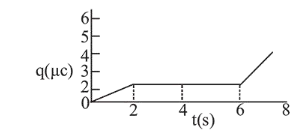# The charge on a capacitor plate in a circuit, as a function of time, is shown in the figure:

Question:

The charge on a capacitor plate in a circuit, as a function of time, is shown in the figure:What is the value of current at $\mathrm{t}=4 \mathrm{~s}$ ?

1. (1) Zero

2. (2) $3 \mu \mathrm{A}$

3. (3) $2 \mu \mathrm{A}$

4. (4) $1.5 \mu \mathrm{A}$

Correct Option: 1

Solution:

(1) Clearly, from graph

Current, I $=\frac{\mathrm{dq}}{\mathrm{dt}}=0$ at $\mathrm{t}=4 \mathrm{~s}$ [Since $\mathrm{q}$ is constant]